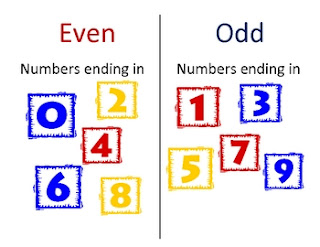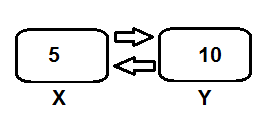## Print all odd number using c programmingOdd and Even number in c
Before doing the code to find all odd number using c programming, we have to know the logic behind the code. We have discussed the logical part. Now it's time to write some code. But I suggest, please go back to the logical part of the code first. The logical thinking to find odd and even number is the same.

## Print all even number using c programming

Before doing code to find all even number using c programming, we have to know the logic behind the code. We have discuss about the logical part. Now it's time to write some code. But I suggest, please go back to the logical part of the code first.Even and Odd number

## Print all even number in c programming

To print all even number from a range in c programming, we have to check all number between given range. To check if the number is odd or even number, we can use this following logic:
The rule is, if any number is fully divided by 2, then this number is even. Else the number is odd.Even and Odd

#### For example

If we want to check whether the number 5 odd or even, we need to simply divide the number by 2.
If there is any reminder left, or if there is any value of 5 mod 2 left, that means 5 is not divisible by 2. So 5 is odd number.

## How to swap 2 number in c programming?

We have discuss about the logic behind this code to solve our problem. If you missed the basic logical part, please go back and understand the logic and then try to code yourself.Swap two number

# How to swap 2 number ?

There is two number in two variable. We have to swap the position of the numbers. For example, theSwap
variable x holding integer value 5 and another variable y holding the integer value 10. Now we have to swap the position of those two numbers.